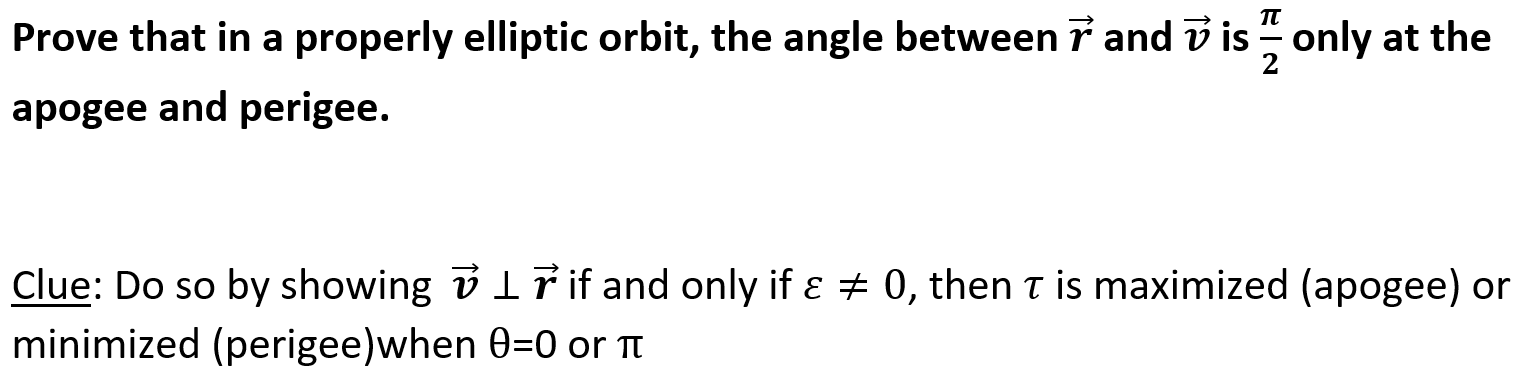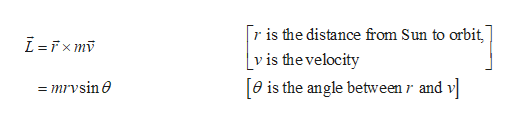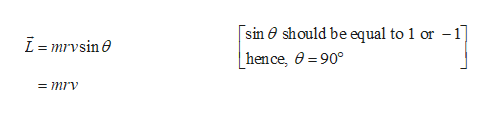properly elliptic orbit, the angle between 7 and v is only at theProve that in a2apogee and perigee.Clue: Do so by showing v ir if and only if a0, then T is maximized (apogee) orminimized (perigee)when 0=0 or TT

Question

I need help with calculus question that deals with physics and elliptical orbits.help_outlineImage Transcriptioncloseproperly elliptic orbit, the angle between 7 and v is only at the Prove that in a 2 apogee and perigee. Clue: Do so by showing v ir if and only if a 0, then T is maximized (apogee) or minimized (perigee)when 0=0 or TT fullscreen
Step 1

Apogee is the farthest point from Sun and Perigee is the nearest point.

Consider the motion of Earth around Sun in elliptical orbit. The direction of velocity is tangential to the elliptical path. The angular momentum about Sun is conserved.

Write the expression for angular momentum.help_outlineImage Transcriptioncloser is the distance from Sun to orbit [v is thevelocity e is the angle between r and v mrvsine fullscreen
Step 2

The radial distance and centripetal force are parallel to each other, hence the torque will be zero.

Step 3

Thus angular momentum will be...help_outlineImage Transcriptionclose[sin 0 should be equal to 1 or -1 L=mrvsine | hen ce, =90° = mrv fullscreen

Want to see the full answer?

See Solution

Want to see this answer and more?

Our solutions are written by experts, many with advanced degrees, and available 24/7

See Solution
Tagged in

Science